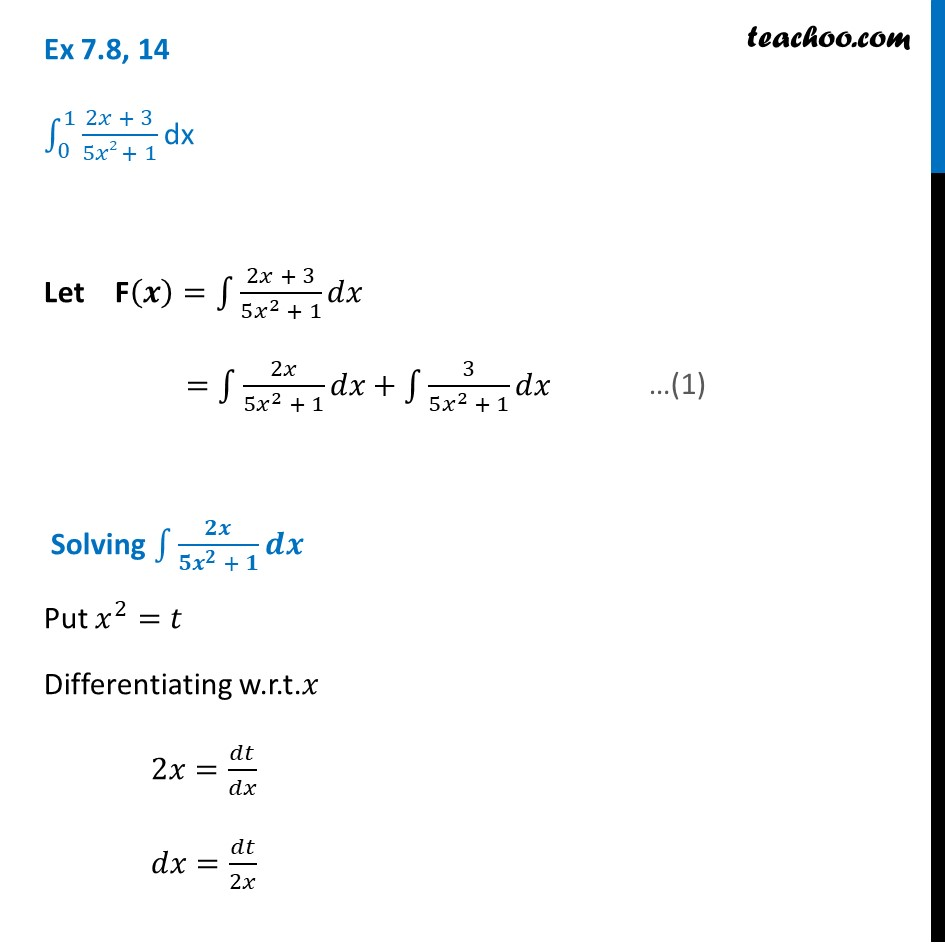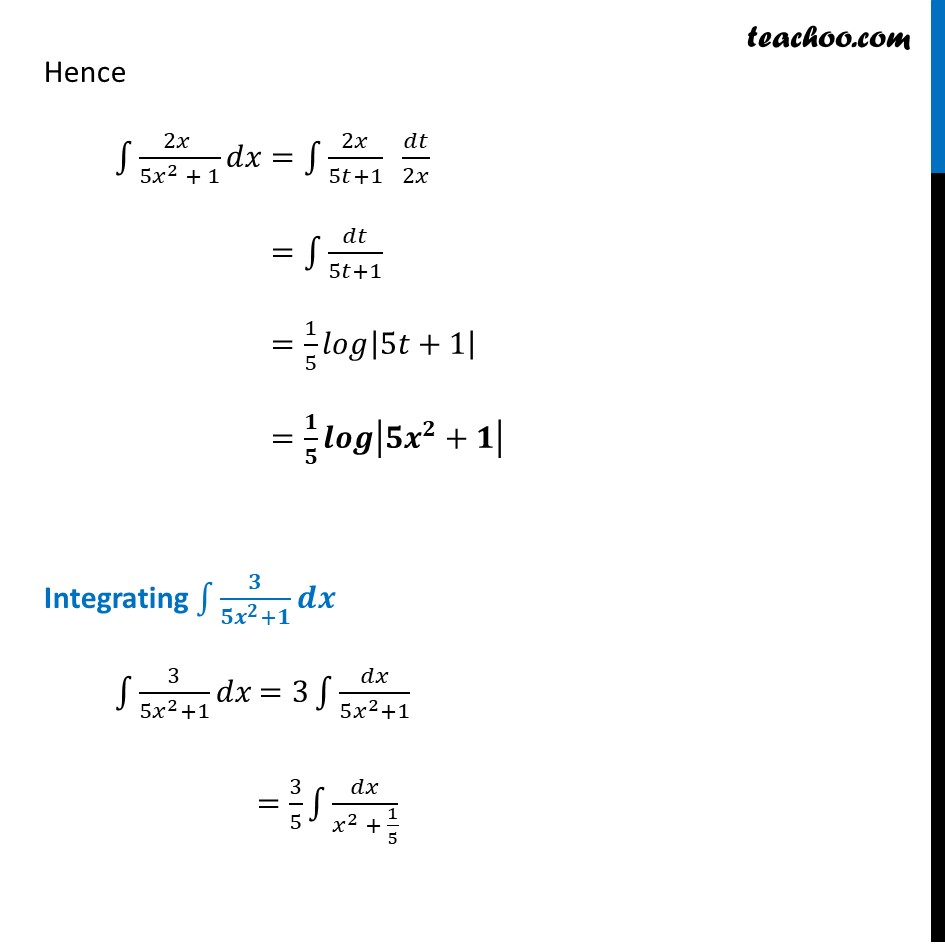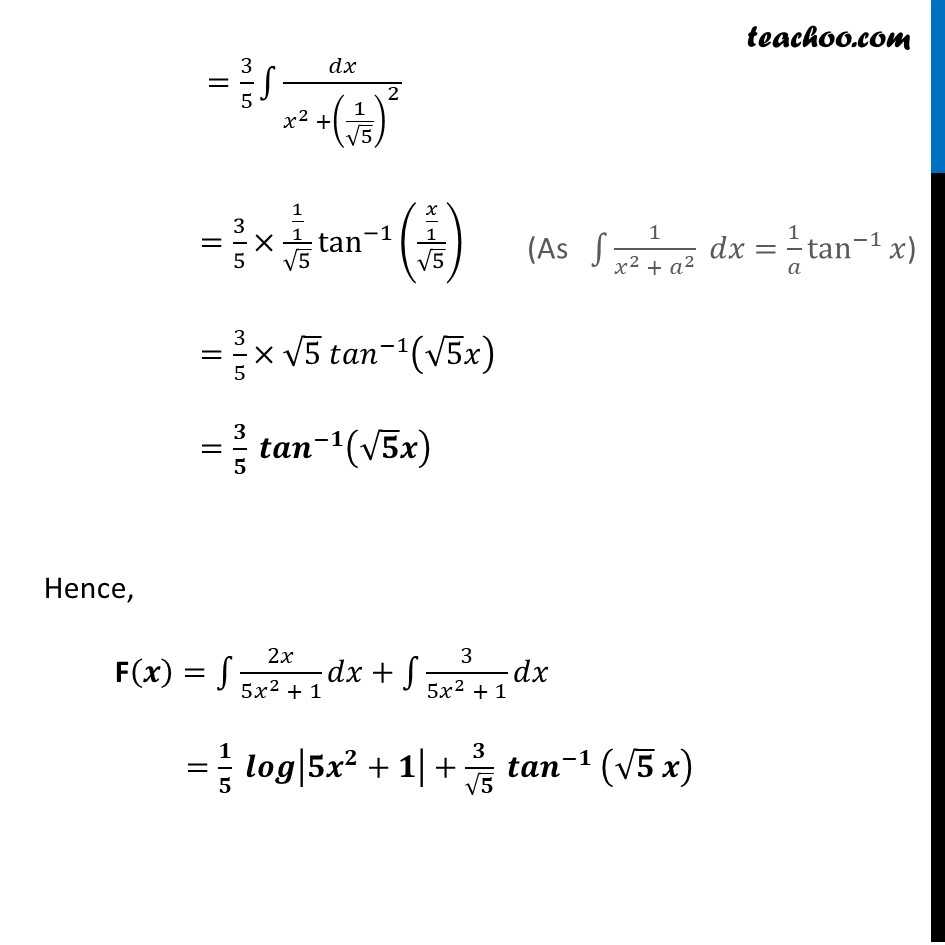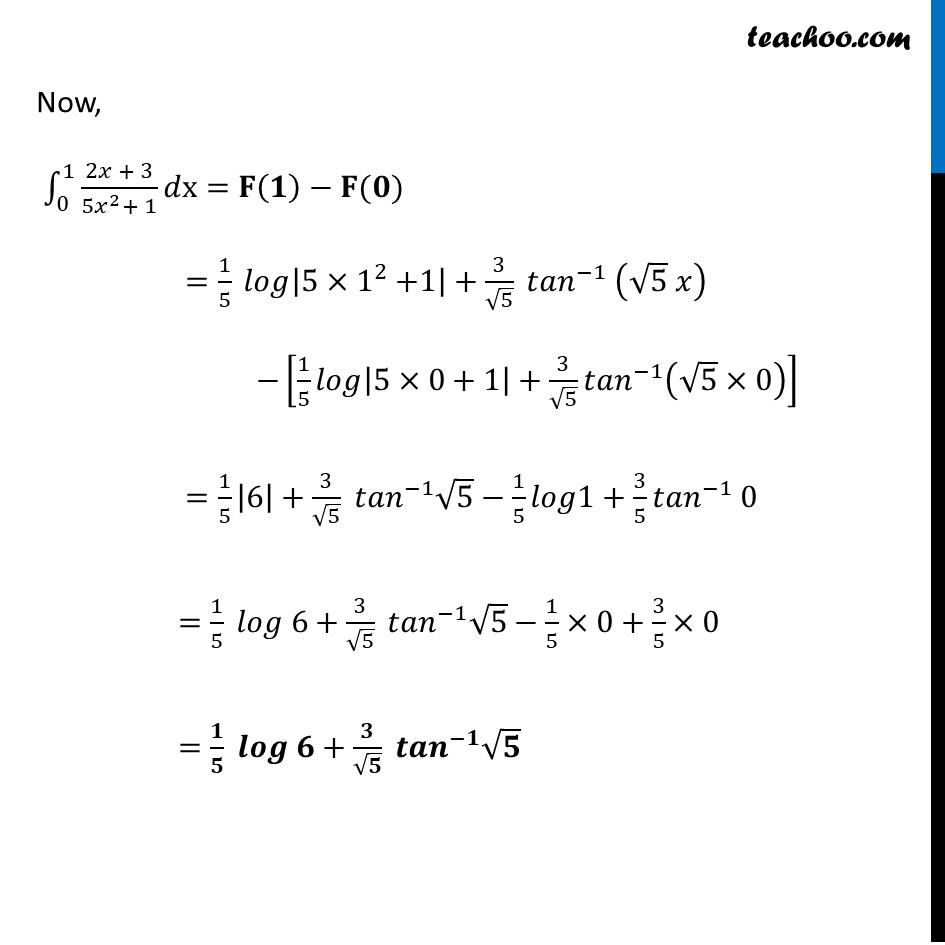Ex 7.8

Chapter 7 Class 12 Integrals
Serial order wiseLearn in your speed, with individual attention - Teachoo Maths 1-on-1 Class

### Transcript

Ex 7.8, 14 ∫_0^1▒(2𝑥 + 3)/(5𝑥2 + 1) dx Let F(𝒙)=∫1▒(2𝑥 + 3)/(5𝑥^2 + 1) 𝑑𝑥 =∫1▒2𝑥/(5𝑥^2 + 1) 𝑑𝑥+∫1▒3/(5𝑥^2 + 1) 𝑑𝑥 Solving ∫1▒𝟐𝒙/(𝟓𝒙^𝟐 + 𝟏) 𝒅𝒙 Put 𝑥^2=𝑡 Differentiating w.r.t.𝑥 2𝑥=𝑑𝑡/𝑑𝑥 𝑑𝑥=𝑑𝑡/2𝑥 Hence ∫1▒〖(2𝑥 )/(5𝑥^2 + 1) 𝑑𝑥=∫1▒〖2𝑥/(5𝑡+1) 𝑑𝑡/2𝑥〗〗 =∫1▒𝑑𝑡/(5𝑡+1) =1/5 𝑙𝑜𝑔|5𝑡+1| =𝟏/𝟓 𝒍𝒐𝒈|𝟓𝒙^𝟐+𝟏| Integrating ∫1▒〖𝟑/(𝟓𝒙^𝟐+𝟏) 𝒅𝒙〗 ∫1▒〖3/(5𝑥^2+1) 𝑑𝑥〗 =3∫1▒𝑑𝑥/(5𝑥^2+1) =3/5 ∫1▒𝑑𝑥/(𝑥^2 + 1/5) =3/5 ∫1▒𝑑𝑥/(𝑥^2 +(1/√5)^2 ) =3/5×(1/1)/√5 tan^(−1) ((𝑥/1)/√5) =3/5×√5 〖𝑡𝑎𝑛〗^(−1) (√5 𝑥) =𝟑/𝟓 〖𝒕𝒂𝒏〗^(−𝟏) (√𝟓 𝒙) Hence, F(𝒙)=∫1▒2𝑥/(5𝑥^2 + 1) 𝑑𝑥+∫1▒3/(5𝑥^2 + 1) 𝑑𝑥 =𝟏/𝟓 𝒍𝒐𝒈|𝟓𝒙^𝟐+𝟏|+𝟑/√𝟓 〖𝒕𝒂𝒏〗^(−𝟏) (√𝟓 𝒙) Now, ∫_0^1▒〖(2𝑥 + 3)/(〖5𝑥〗^2+ 1) 𝑑x=𝐅(𝟏)−𝐅(𝟎)〗 =1/5 𝑙𝑜𝑔|5〖×1〗^2+1|+3/√5 〖𝑡𝑎𝑛〗^(−1) (√5 𝑥) −[1/5 𝑙𝑜𝑔|5×0+1|+3/√5 〖𝑡𝑎𝑛〗^(−1) (√5×0)] =1/5 |6|+3/√5 〖𝑡𝑎𝑛〗^(−1) √5−1/5 𝑙𝑜𝑔1+3/5 〖𝑡𝑎𝑛〗^(−1) 0 =1/5 𝑙𝑜𝑔 6+3/√5 〖𝑡𝑎𝑛〗^(−1) √5−1/5×0+3/5×0 =𝟏/𝟓 𝒍𝒐𝒈 𝟔+𝟑/√𝟓 〖𝒕𝒂𝒏〗^(−𝟏) √𝟓